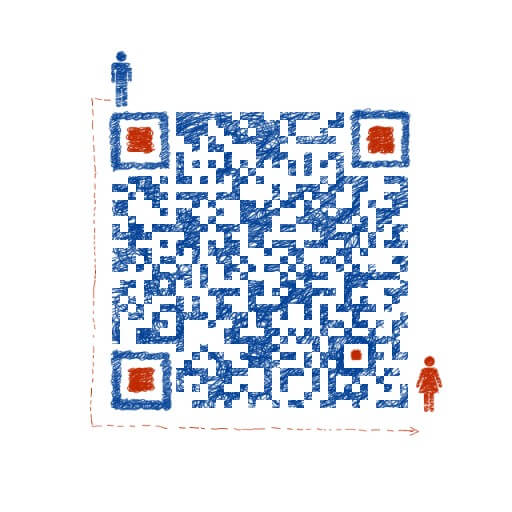Javascript|

# 温故js系列（1）

## 基本数据类型和引用数据类型判断 & 存储访问 & 类型转换

Posted by xzavier on July 3, 2016

## 数据类型定义

### 数据类型分类

js的常见内置对象：`Date,Array,Math,Number,Boolean,String,Array,RegExp,Error,Function...`

### 数据类型访问&&复制

``````var a = 1;
````````````var b = a;
````````````b = 2;
````````````var a = new Object();
````````````a.name = 'xz'; ![图片描述]
``````

``````var b = a;
````````````b.sex = 'boy';
````````````b.sex;  //'boy'   a.name; //'boy'
``````

## 数据类型检测

### Typeof

typeof操作符是检测基本类型的最佳工具。

``````"undefined" — 未定义

"boolean"   — 布尔值

"string"    — 字符串

"number"    — 数值

"object"    — 对象或null

"function"  — 函数
``````

### Instanceof

instanceof用于检测引用类型，可以检测到它是什么类型的实例。

instanceof 检测一个对象A是不是另一个对象B的实例的原理是：查看对象B的prototype指向的对象是否在对象A的[[prototype]]链上。如果在，则返回true,如果不在则返回false。不过有一个特殊的情况，当对象B的prototype为null将会报错(类似于空指针异常)。

``````var sXzaver = new String("Xzavier");
console.log(sXzaver instanceof String);   //  "true"
var aXzaver = [1,2,3];
console.log(aXzaver instanceof Array);   //  "true"

``````

constructor属性返回对创建此对象的数组函数的引用。可以用于检测自定义类型。

``````'xz'.constructor == String // true
(123).constructor == Number // true
(true).constructor == Boolean // true
[1,2].constructor == Array // true
({name:'xz'}).constructor == Object // true
(function(){}).constructor == Function // true
(new Date()).constructor == Date // true
(Symbol()).constructor == Symbol // true
(/xz/).constructor == RegExp // true
``````

constructor不适用于null和undefined。除了这些原生的，constructor还可验证自定义类型。

``````function Xzavier(){}
var xz = new Xzavier();
xz.constructor == Xzavier;  // true
``````

### Object.prototype.toString.call(obj)

``````原理：调用从Object继承来的原始的toString()方法

Object.prototype.toString.call('xz'); //"[object String]"
Object.prototype.toString.call(123);  //"[object Number]"
Object.prototype.toString.call(true); //"[object Boolean]"
Object.prototype.toString.call([1,2]); //"[object Array]"
Object.prototype.toString.call({name:'xz'}); //"[object Object]"
Object.prototype.toString.call(function(){}); //"[object Function]"
Object.prototype.toString.call(null); //"[object Null]"
Object.prototype.toString.call(undefined); //"[object Undefined]"
Object.prototype.toString.call(); //"[object Undefined]"
Object.prototype.toString.call(new Date()); //"[object Date]"
Object.prototype.toString.call(/xz/);  //"[object RegExp]"
Object.prototype.toString.call(Symbol()); //"[object Symbol]"

var obj = {name:"Xzavier", age:23};
var a = [1,2,3];

function isType(obj) {
return Object.prototype.toString.call(obj).slice(8, -1);
}
isType(obj);  // "Object"
isType(a)  // "Array"
``````

## 数据类型转换

### 隐式转换

``````undefined == null;  // true
1 == true;  // true
2 == true;  // false
0 == false;  // true
0 == '';  // true
NaN == NaN;  // false  NaN不等于任何值
[] == false;  // true
[] == ![];  // true
'6' - '3'  // 3
1234 + 'abcd' // "1234abcd"
``````

1.undefined与null相等，但不恒等（===）

2.一个是number一个是string时，会尝试将string转换为number

3.隐式转换将boolean转换为number，0或1

4.隐式转换将Object转换成number或string，取决于另外一个对比量的类型

5.对于0、空字符串的判断，建议使用 “===” 。

6.“==”会对不同类型值进行类型转换再判断，“===”则不会。它会先判断两边的值类型，类型不匹配时直接为false。

### 显示转换

#### Number：

``````Number('1234') // 1234
Number('1234abcd') // NaN
Number('') // 0
Number(true) // 1
Number(null) // 0
Number(undefined) // NaN
``````

#### String：

``````String(1234)  // "1234"
String('abcd')  // "abcd"
String(true)  // "true"
String(undefined) // "undefined"
String(null)  // "null"
``````

#### Boolean:

``````Boolean(0)  // false
Boolean(undefined)  // false
Boolean(null)  // false
Boolean(NaN)  // false
Boolean('')  // false
``````

``````!!'foo';   // true
!!'';      // false
!!'0';     // true
!!'1';     // true
!!'-1'     // true
!!{};      // true
!!true;    // true
``````

Number、String、Boolean转换对象时主要使用了对象内部的valueOf和toString方法进行转换。

#### Number转换对象：

1.先调用对象自身的valueOf方法。如果返回原始类型的值，则直接对该值使用Number函数，返回结果。

2.如果valueOf返回的还是对象，继续调用对象自身的toString方法。如果toString返回原始类型的值，则对该值使用Number函数，返回结果。

3.如果toString返回的还是对象，报错。

``````Number(); //1

.valueOf(); // ;
.toString(); // '1';
Number('1'); //1
``````

#### String转换对象

1.先调用对象自身的toString方法。如果返回原始类型的值，则对该值使用String函数，返回结果。

2.如果toString返回的是对象，继续调用valueOf方法。如果valueOf返回原始类型的值，则对该值使用String函数，返回结果。

3.如果valueOf返回的还是对象，报错。

``````String([1,2]) //"1,2"

[1,2].toString();  //"1,2"
String("1,2");  //"1,2"
``````

#### Boolean转换对象

Boolean转换对象很特别，除了以下六个值转换为false，其他都为true

``````undefined  null  false  0(包括+0和-0)  NaN  空字符串('')
Boolean(undefined)   //false
Boolean(null)        //false
Boolean(false)       //false
Boolean(0)           //false
Boolean(NaN)         //false
Boolean('')          //false

Boolean([])          //true
Boolean({})          //true
Boolean(new Date())  //true
``````